Machine Learning Workflow using Pycaret

• Last Updated : 11 May, 2021

PyCaret is an open-source machine learning library which is simple and easy to use. It helps you right from the start of data preparation to till the end of model analysis and deployment. Moreover, it is essentially a python wrapper around several machine learning libraries and frameworks such as scikit-learn, spaCy etc, It also has the support of complex machine learning algorithms which are tedious to tune and implement.So why to use Pycaret. Well, there are lots of reasons for this let me explain to you a few of them. The first Pycaret is a low-code library which makes you more productive while solving a business problem. Second Pycaret can do data preprocessing and feature engineering with a single line of code, where in reality, it is very time-consuming. Third Pycaret allows you to compare different machine learning models and finetune your model very easily. Well, there are many other advantages but for now, stick with them.

Installation

pip install pycaret

if you are using Azure Notebooks or Google Colab

!pip install pycaret

Let’s start by importing required libraries.

Python3

 # importing required libraries# for reading and manipulating dataimport numpy as npimport pandas as pd

Reading the dataset using pandas library

Python3

Output: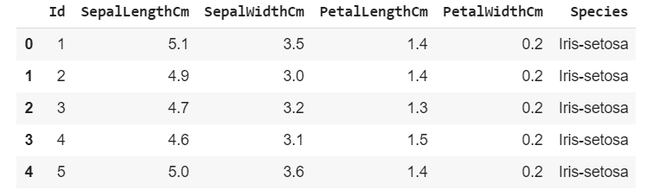Starting with pycaret

Initializing the setup

Python3

 #import classification module from pycaretfrom pycaret.classification import * #intialize the setupclf = setup(iris_classification, target = 'Species')

setup takes our data iris_classification and the target value(which needs to predicted) in our case it is Species

Output: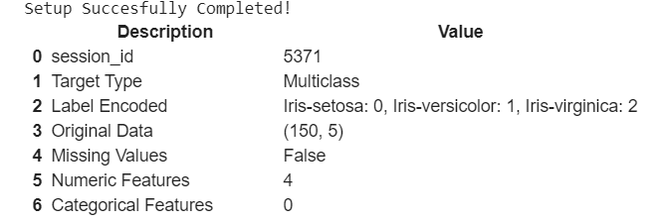compressed output

It gives basic description of our dataset, you can see it automatically encoded the target variables into 0,1,2.

Now let’s compare various classification models that Pycaret built for us

Python3

 # comparing different# classification modelscompare_models()

Output: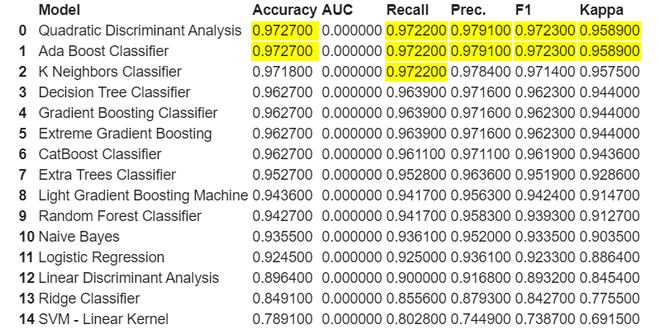As we can see here it highlights the highest value in each respective column. Here for this classification both Quadratic Discriminant Analysis and Ada Boost Classifier both are performing well let’s take QDA for our further model creation and analysis.

Creation of model

Python3

 # creating model qdamodel = create_model('qda')

Output: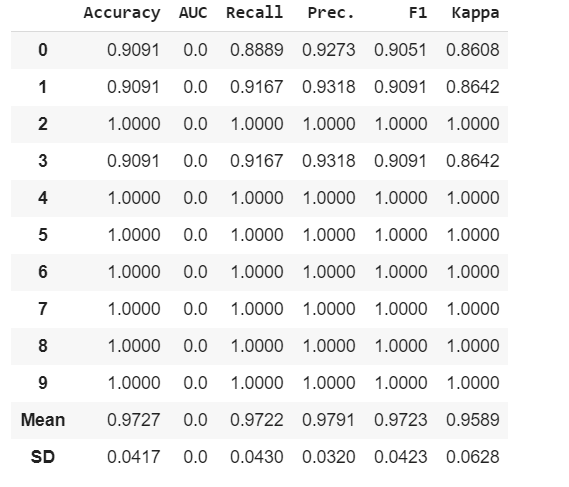It shows various metrics used to evaluate model on different folds.

Let’s tune the model hyperparameters

Python3

 # tuning model hyperparameterstuned_model = tune_model('qda')

Output: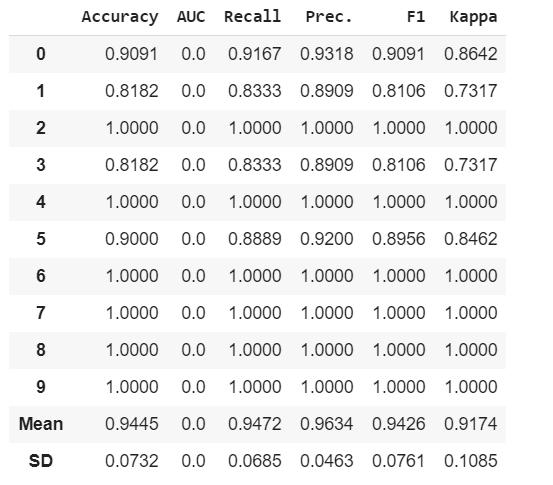We can see here some Recall, Precision, F1 and Kappa has increased because of fine tuning of our model.

Now let’s do some model analysis

Python3

 # plotting boundaries between different# labelsplot_model(tuned_model, plot = 'boundary')

Output: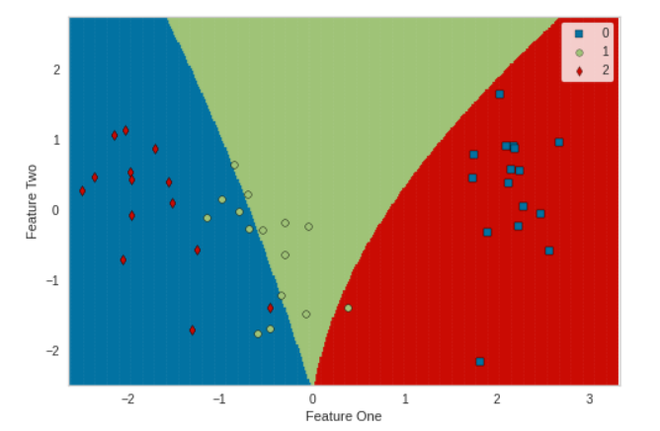Python3

 # plotting confusionmatrix for predicted labelsplot_model(tuned_model, plot = 'confusion_matrix')

Output: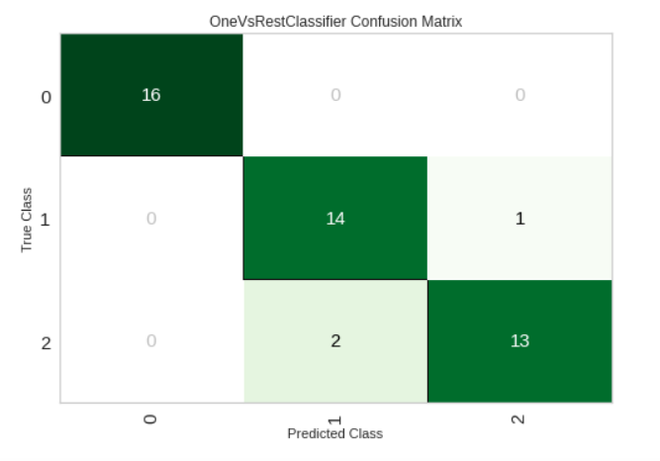Python3

 # plotting number of correctly# classified and misclassifed labelsplot_model(tuned_model, plot = 'error')

Output: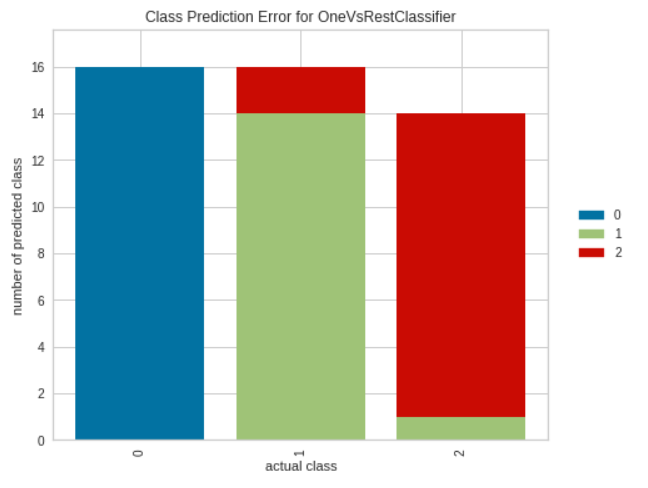Python3

 # plotting classification reportplot_model(tuned_model, plot = 'class_report')

Output: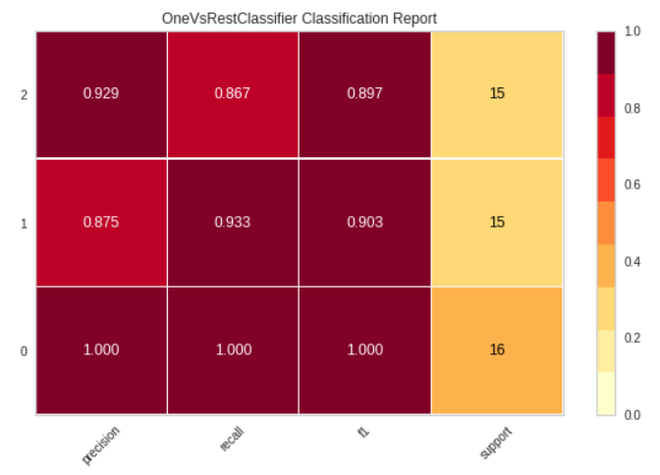Finalize the model

Python3

 # finalizing the tuned_modelfinalize_model(tuned_model)

Output: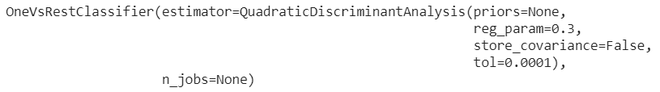Saving the model

Python3

 # saving the modelsave_model(tuned_model, 'qda1')

Output: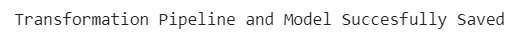Attention geek! Strengthen your foundations with the Python Programming Foundation Course and learn the basics.

To begin with, your interview preparations Enhance your Data Structures concepts with the Python DS Course. And to begin with your Machine Learning Journey, join the Machine Learning - Basic Level Course

My Personal Notes arrow_drop_up